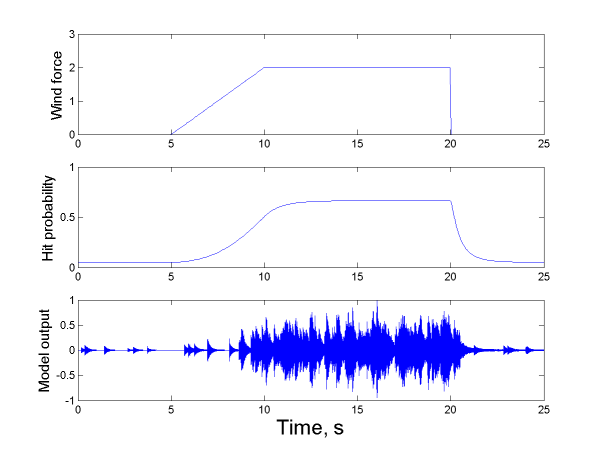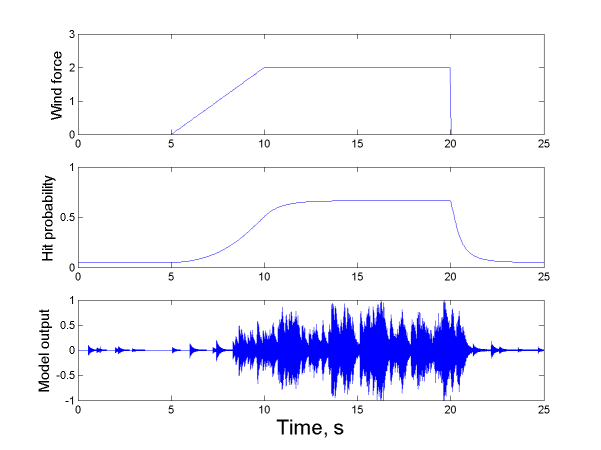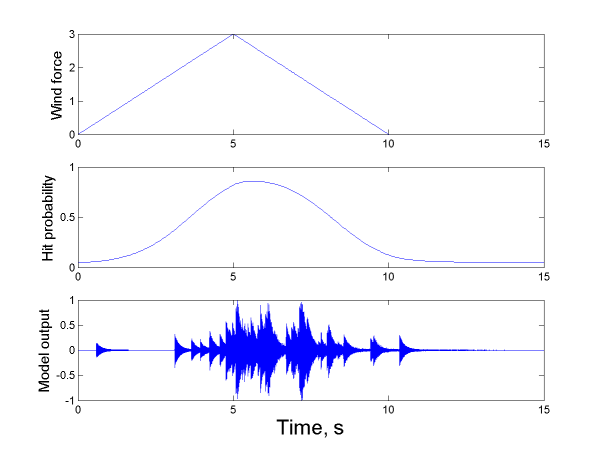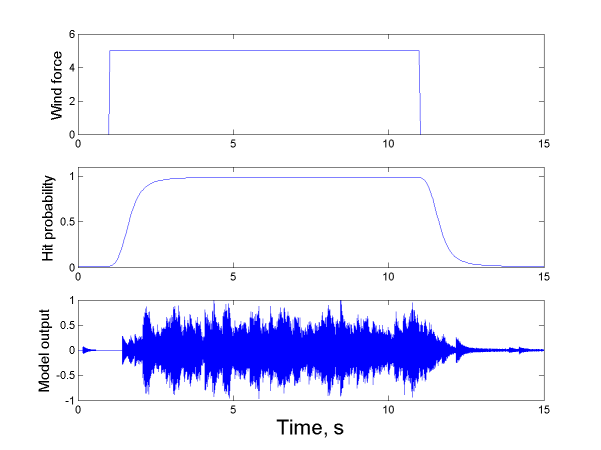# Modal Synthesis of Wind Chime Sounds with Stochastic Event Triggering

## Introduction

This page presents sound examples generated with the wind chime model descibed in the paper with the same title published in the proceedings of the NORSIG04 conference:
"Modal Synthesis of Wind Chime Sounds with Stochastic Event Triggering" (PDF)

The sound sample used in the parameter extraction process was taken from http://www.101windchimes.com/. The particular sample we used is here.

## Sound Example Discussed in the Article

The example given in the article. All the images below show the wind force function (top), hit probability (middle) and model output (bottom) as functions of time.Another example generated with exactly the same wind force function. It is similar to, but not identical with, the previous example. This is due to the stochastic nature of the model.## Other Examples

An example with a triangular wind force function.An example where the wind is very strong. Note that the scale on the y-axis of the top figure is not the same as in the other wind force function plots.